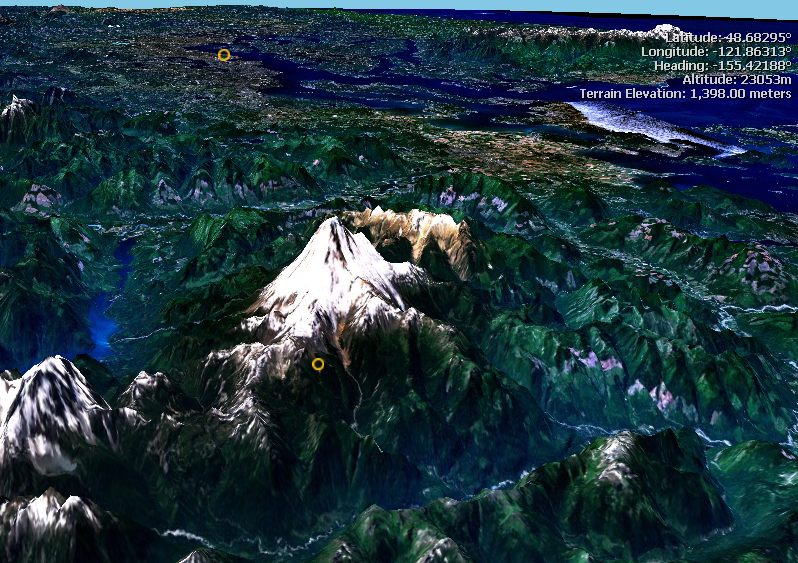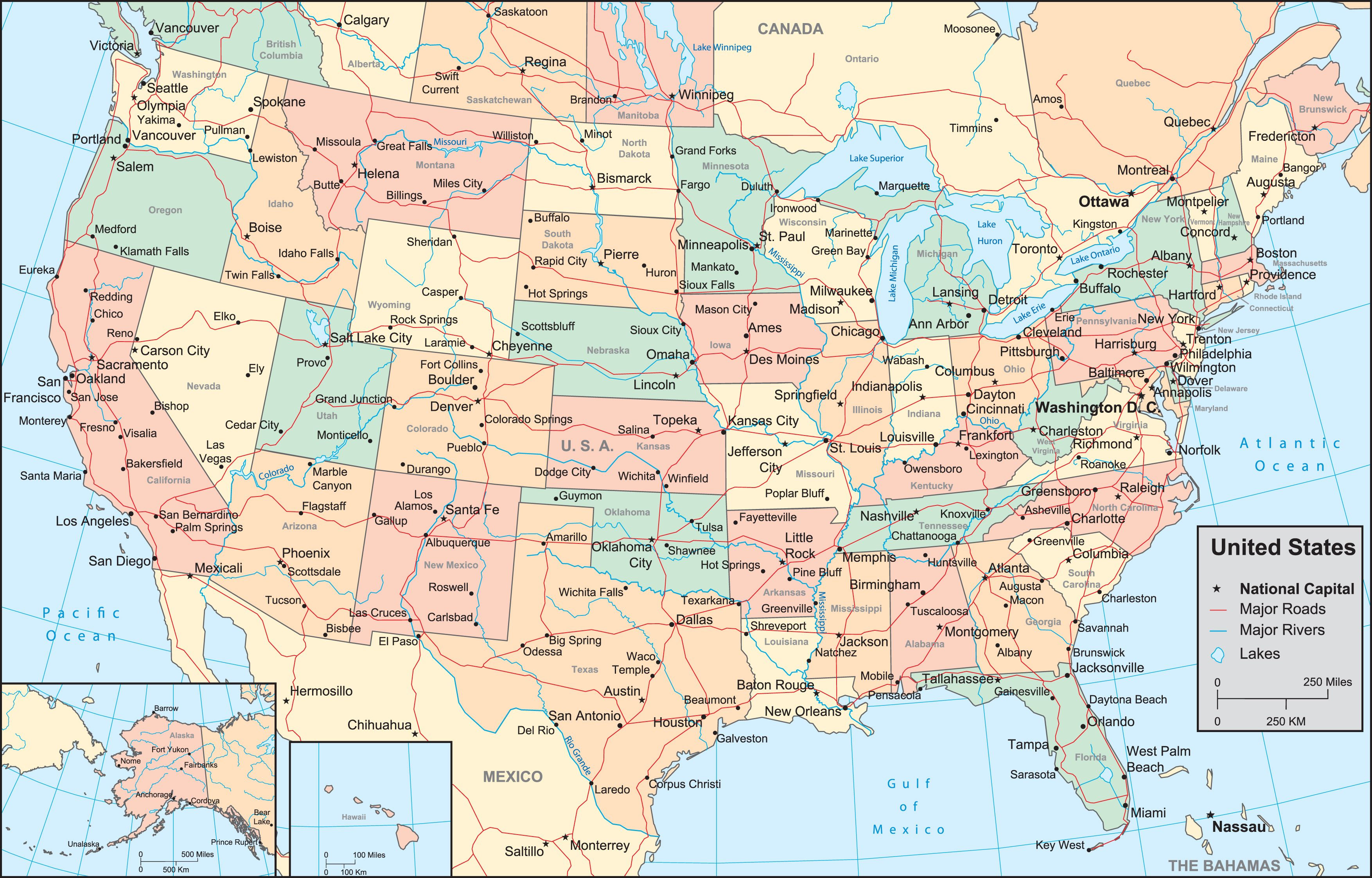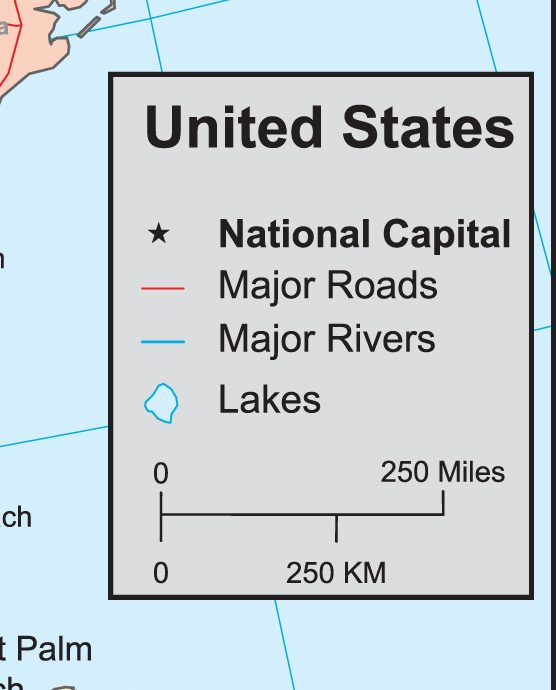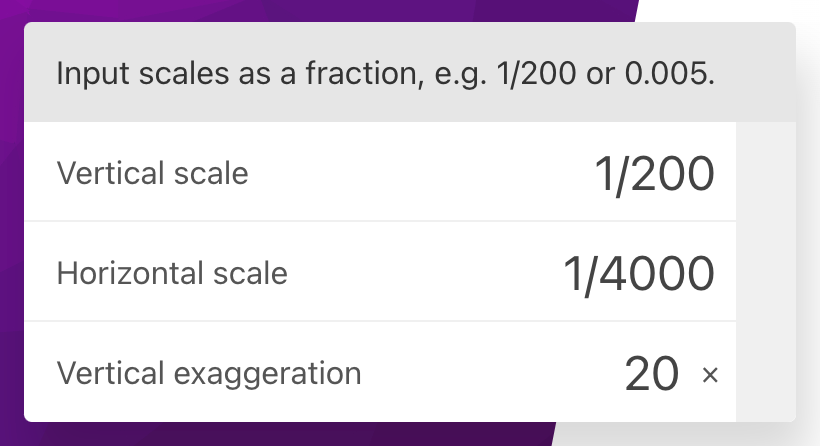# Vertical Exaggeration Calculator

Created by Antoni Kępiński
Reviewed by Hanna Pamuła, PhD and Jack Bowater
Last updated: Jan 03, 2023

Welcome to our vertical exaggeration calculator! Let's assume you would like to create a raised-relief map or a three-dimensional drawing/animation. If so, it's essential to consider the vertical exaggeration, as ignoring it may lead to your work being unreadable to some viewers. Don't worry, though - this article will explain precisely how the vertical exaggeration formula works and, most importantly, what are its practical uses.

In the end, you will also learn the meaning of other terms, such as the vertical scale and horizontal scale, and how they connect with the equation mentioned earlier.

Are you rendering a three-dimensional animation? Check out our 3d render calculator to estimate the rendering time!

## What is a vertical exaggeration?

Vertical exaggeration is a scale used primarily in the field of geography but also in engineering. It emphasizes certain terrain features or objects by making them larger, ergo increasing their overall visibility. It all sounds overly complicated, so let's bring some visualizations to make things more straightforward.The image above shows a computer-rendered aerial view of Mount Baker, an active stratovolcano in Washington, U.S.A. While you probably wouldn't be able to tell, it has been vertically exaggerated, meaning in the real world, it would appear much flatter.

So, to summarize, vertical exaggeration allows us to increase the readability of raised-relief (three-dimensional) maps and technical drawings by modifying the height of certain areas or objects.

## What is the vertical exaggeration formula?

The vertical exaggeration formula is not that complicated:

VE = VS / HS

Where:

• VE - Vertical exaggeration, given as a number, e.g. 5× or 20×;
• VS - Vertical scale, given as a fraction, e.g. 1 : 200 (same as 1 / 200); and
• HS - Horizontal scale, same as above.

As you see, to calculate the vertical exaggeration used in a map, we first need to know its vertical and horizontal scale. The scale is a fundamental property of every geographical map and can usually be found in the map legend:In this case, the legend is positioned just a bit above the bottom right corner:Most maps nowadays tend to use a linear scale, also known as bar or graphic scale. Assuming the distance measured by a ruler from "0" to "250 KM" is 1 centimeter, the representational scale of the map is 1 : 25M (centimeters).

We highly recommend our scale calculator, which you can use to quickly calculate either the horizontal scale or the vertical scale, both of which you can then use in the vertical exaggeration formula. Alternatively, you can also click on the advanced mode button.

## How to calculate vertical exaggeration?

The easiest way to calculate vertical exaggeration is by using our free calculator on this page! You only need to supply 2 of the elements from the formula presented above.

For example, assuming you want to get the vertical exaggeration of a 3D map with a vertical scale of 1 : 200 and a horizontal scale of 1 : 4000:Here is how you should interpret the result:

• A value higher than 1× indicates vertical exaggeration, e.g., 10× means that vertical measurements are ten times greater than horizontal measurements;
• A value equal to 1× implies no vertical exaggeration; and
• A value lower than 1× means horizontal exaggeration, the opposite of vertical exaggeration.

As you can see, you don't need to be an expert to understand the concept of vertical exaggeration, how to calculate it, and where you can use it. We hope that you found this calculator helpful!

Antoni Kępiński
Input scales as a fraction, e.g. 1/200 or 0.005.
Vertical scale
Horizontal scale
Vertical exaggeration
×
People also viewed…

### CSS aspect ratio

Our CSS aspect ratio calculator allows you to quickly resize your files to fit in a designated area.

### Chilled drink

With the chilled drink calculator you can quickly check how long you need to keep your drink in the fridge or another cold place to have it at its optimal temperature. You can follow how the temperature changes with time with our interactive graph.

### Titration

Use our titration calculator to determine the molarity of your solution.

### Total harmonic distortion

The total harmonic distortion calculator is a tool that converts signal distortion from percent to decibel value and vice versa.